Deformation mechanisms in negative Poisson's ratio materials: structural aspects
adapted from J. Materials Science, 26, 2287-2292 (1991).

 Poisson main   Original article   Chirality   Synthesis method   Names   Advances   Structural hierarchy End effects   Fastener   Work by others

Preface
Negative Poisson's ratio material expands laterally when stretched, in contrast to ordinary materials. Negative Poisson's ratio foam was first developed by Rod Lakes. Later writers have called such materials anti-rubber, auxetic materials, auxetics, or dilatational materials. Here we study the causal mechanisms responsible for the negative Poisson's ratio. We remark that the role of phase transformations and of structure size is explored elsewhere.

Abstract
Poisson's ratio in materials is governed by the following aspects of the microstructure: the presence of rotational degrees of freedom, non-affine deformation kinematics, or anisotropic structure. Several structural models are examined. The non-affine kinematics are seen to be essential for the production of negative Poisson's ratios for isotropic materials containing central force linkages of positive stiffness. Non-central forces combined with pre-load can also give rise to a negative Poisson's ratio in isotropic materials. A chiral microstructure with non-central force interaction or non-affine deformation can also exhibit a negative Poisson's ratio. Toughness and damage resistance in these materials may be affected by the Poisson's ratio itself, as well as by generalized continuum aspects associated with the microstructure.

1. Introduction
A new class of cellular materials was reported by the author ; these materials exhibit a negative Poisson's ratio. Such materials expand laterally when stretched and contract laterally when compressed. This unusual characteristic is achieved by forming the cells into a 're-entrant' shape which bulges inwards and which unfolds under tension resulting in a lateral expansion. These cellular solids can be made from a variety of polymers  or from metals [1,2]. Negative Poisson's ratio materials (called auxetic by Evans and others) are of interest because of their unusual nature, and because they deform in ways unexpected on the basis of experience with ordinary materials. For example, their shear modulus can substantially exceed their bulk modulus, a situation opposite that seen in rubbery materials. Moreover, many relationships for deformation in the theory of elasticity contain terms (1 - [[nu]]^2) with [[nu]] as Poisson's ratio, so that for [[nu]] approaching its lower limit -1, enhanced shear rigidity or high toughness can be achieved , which can be of use in various applications . The materials also exhibit unusual acoustic properties related analytically to the Poisson's ratio [4,5], as well as acoustic properties experimentally determined and related to the re-entrant structure directly .

Negative Poisson's ratios can also arise in two-dimensional honeycombs with inverted cells , in rocks with microcracks [8,9], in an anisotropic microcellular polymer, expanded polytetrafluoroethylene [10,11], in anisotropic fibrous media in some directions[12-14], in anisotropic pyrolytic carbon . While it had been reported that single crystal pyrites had a negative Poisson's ratio, recent study indicates otherwise . Thin (anisotropic) magnetized ferromagnetic films have been reported to exhibit a transient negative Poisson's ratio which disappears with aging . Macroscopic structures of sliders, hinges, and springs can also exhibit negative Poisson's ratio . A variety of structural models can give rise to a negative Poisson's ratio , nevertheless negative Poisson's ratios are unusual. Indeed, in a large compilation of properties of polycrystalline materials , most have Poisson's ratio in the vicinity of 1/3 with exceptions such as beryllium which has a Poisson's ratio of about 0.1, and ammonium chloride which assumes a negative value over a narrow temperature range.

It is the purpose of this article to identify the relevant microstructural features associated with negative Poisson's ratio materials and to present several structural models which exhibit these features in isolation. In the next section the role of structure in determining Poisson's ratio is explored, and in the section following that, the connection between structures of interest and generalized continuum mechanics is examined.

2. Microstructure and Poisson's ratio

2.1 Cauchy relations

In the early development of the theory of elasticity, it was believed by some (such as Navier and Poisson) that isotropic materials were describable by only one elastic constant and that Poisson's ratio was 1/4 for all isotropic materials. The basis for this view (so called uniconstant theory) was an atomic model in which the atoms as point particles in a centrosymmetric lattice  interacted by central forces dependent upon distance alone. Based on such a model, the tensorial elastic constants of an anisotropic solid become related by 'Cauchy relations'; for an isotropic medium, the Cauchy relations imply a Poisson's ratio of 1/4 for all materials described by the model. These arguments have been recapitulated by modern authors [21-23]. Experiments disclosed Poisson's ratios for most common isotropic materials to be close to 1/3, resulting in the abandonment of uniconstant elasticity theory governed by the Cauchy relations. The classical theory of elasticity now used incorporates two independent elastic constants for isotropic materials, and we realize that the above simple model does not have general applicability.

Poisson's ratios differing from 1/4 can arise from deviations from the above assumptions, specifically (i) non-central forces between particles in the solid, (ii) forces which do not depend on distance alone, or (iii) anisotropy, including noncentrosymmetry. We address in this article the question of what kind of interaction results in a Poisson's ratio which is negative, not just different from 1/4. To that end, we consider several microstructures with the aim of distinguishing which kinds of interaction are most important to achieve a negative Poisson's ratio. Most of these structures are two-dimensional and isotropic in plane in their classical elastic properties either by virtue of the choice of the elastic stiffness of the micro-elements or by symmetry. As for symmetry, materials which are structurally hexagonal, are transversely isotropic, i.e. isotropic in plane, within the framework of classical elasticity . The range for Poisson's ratio [[nu]] for isotropic materials is -1< [[nu]] <1/2 in three dimensions and -1< [[nu]] <1 in two dimensions.

Under static conditions, noncentral forces must be acompanied by a moment, to satisfy the equilibrium equations. The kinematical variable conjugate to a moment is a rotation angle. As for forces which do not depend on distance but instead connect only selected pairs of particles, the corresponding kinematical deformation is a non-affine one. This is in contrast to affine deformation in which the particles in the solid move in a way corresponding to a uniform strain plus a rotation in a continuum, ie, uj = ajk xk in which uj is the displacement, xk is the particle position, ajk is a tensor describing the deformation, and repeated indices are summed over. Affine deformation kinematics are necessary conditions for the Cauchy relations to be obtained .

2.2 Non-affine deformation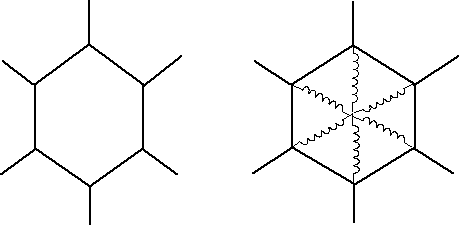Fig. 1 Hexagonal honeycomb structure of bendable ligaments.

A honeycomb composed of regular hexagonal cells (Fig.1) has a Poisson's ratio of +1 [7,25]. The cell ribs undergo bending if the honeycomb is stretched or sheared. The deformation is not affine since some pairs of nodal points move apart during stretching while others do not. Indeed, a honeycomb without any bending can be made of elastic (spring) elements free to rotate at the joints. Since they would rotate without stretching if the honeycomb were under tension, such a structure has a zero Young's modulus. To obtain an elastic honeycomb, additional soft elements could be inserted to supply the restoring force; the Poisson's ratio would be slightly less than 1.

Re-entrant honeycomb cells such as those shown in Fig. 2 give rise to a negative Poisson's ratio [25,26].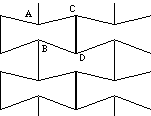Fig. 2 Re-entrant honeycomb with negative Poisson's ratio, made of bendable ligaments. A similar structure can be made with rigid ligaments if a spring is placed between points of type A and B.
The ligaments undergo bending and the deformation is manifestly non-affine in that the cells unfold during stretching of the honeycomb. In particular, points A and B move further apart than expected from the global strain, while points C and D maintain their separation during stretching. Again, bending is not essential in that a similar effect can be achieved with stretchable (spring) elements only, which are free to pivot with no rotational constraint. The structure is orthotropic, however by appropriate choice of the rib widths and angles, an elastically isotropic honeycomb with a Poisson's ratio of -1 can be obtained. For comparison, a structurally hexagonal re-entrant honeycomb structure is shown in Fig. 3; this is isotropic in plane by virtue of symmetry. In this case as well, the bendable ligaments can be replaced by rigid ones which are free to pivot, and the restoring force supplied by elastic elements (not shown).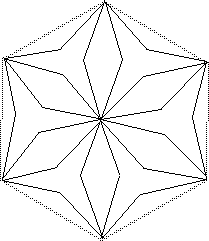Fig. 3 Structurally hexagonal re-entrant honeycomb with negative Poisson's ratio. Solid lines are bendable ribs.

2.3 Non-central forces

The above examples indicate it is possible to obtain large magnitudes of Poisson's ratio, including negative values, using central force interactions alone. These structures deform in a non-affine manner. We now consider the effect of non-central forces alone, with affine deformation. A two-dimensional structure of rigid rotatable nodes linked by elastic ligaments, originally examined in a study of generalized continuum mechanics , is of interest in this vein and is shown in Fig. 4.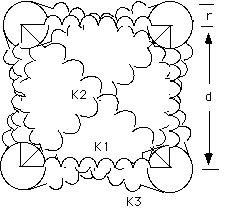Fig. 4 Lattice of rigid rotatable nodes linked by elastic ligaments.

The structure is cubic, however it is possible to obtain elastic isotropy by choice of the stiffnesses of the elastic ligaments. Given the Lamé and Cosserat elastic constants provided in , we invoke isotropy (which results in a relationship between stiffnesses), set up the lattice without prestrain in any of the ligaments, and calculate the engineering elastic constants in terms of the node size and the relative magnitude of the noncentral forces. Calculated Poisson's ratios are shown in Fig. 5.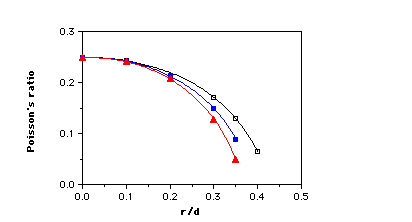Fig. 5 Behavior of structure shown in Fig. 4. Poisson's ratio vs rotatable node size r divided by lattice spacing d, and spring stiffness ratio k3/k1 which is a measure of the relative magnitude of the noncentral forces. Poisson's ratio is positive if all the ligament stiffnesses are positive.

Open squares, k3/k1 =1 Solid squares, k3/k1 = 2.

Triangles, k3/k1 = 5. +, k3/k1 =1/2.

Observe that a rotatable node size of zero or a zero stiffness for the ligaments k3 (which are attached to the rotatable node periphery) results in a lattice governed by purely central forces; the Poisson's ratio is 1/4 as is the case in three dimensions. Introduction of non-central forces reduces the Poisson's ratio, however negative Poisson's ratios are not obtained unless one of the ligament stiffnesses becomes negative. Such a ligament would be unstable if isolated, but the stability criterion for the entire lattice remains -1< [[nu]] < 1. If the restriction of isotropy in this structure is relaxed, the minimum Poisson's ratio is still zero for nonnegative ligament stiffness. The structure in Fig. 4 may be considered in relation to an earlier analytical study of granular materials . Negative Poisson's ratios are theoretically possible in such granular systems if the stiffness for tangential deformation were to exceed that for normal deformation. However for spherical granules with perfect slip, [[nu]] = 1/4, while for contact without slip, 0 <= [[nu]] <= 0.11, consequently Poisson's ratio is positive for real granular materials.

Non-central forces can also be introduced by endowing the lattice's connecting ligaments with bending and torsional rigidity. We consider such a three-dimensional cubic lattice of points linked by extendable, bendable and twistable ribs . Given arbitrary rib stiffnesses, the structure is orthotropic, however we derive conditions on the ligament stiffnesses to achieve isotropy. We find the Poisson's ratio to be 1/4 for zero rib bend stiffness. The bend rigidity of straight ribs reduces the Poisson's ratio by a small amount even if the ribs are thick. The lower bound on Poisson's ratio for this lattice is zero, and it is approached if the bend stiffness of the ribs can be made larger than the extensional stiffness.

For the structure in Fig. 4, let us introduce a prestrain in the vertical and horizontal ligaments k1. The corresponding prestrain is then determined for the ligaments k3 based on equilibrium considerations. Ligament stiffness is again kept positive. Poisson's ratio can be made either greater or less than 1/4 or can be made negative as shown in Fig. 6.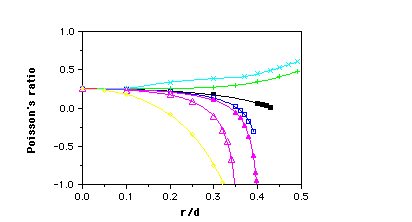Fig. 6 Poisson's ratio vs rotatable node size r divided by lattice spacing d, for non-central force lattice shown in Fig. 4, with prestrain in the ligaments. Prestrain factor f is the ratio of natural length of the vertical and horizontal ligaments to their length in the lattice, the lattice spacing. k3/k1 is a ratio of ligament stiffnesses.

Solid squares, k3/k1 = 1, f =1.
Open squares, k3/k1 = 1/2, f =1/2.
Solid triangles, [[Delta]] k3/k1 = 1/4, f =1/2
Open triangles, [[Delta]] k3/k1 = 1/8, f = 1/8
Diamonds, [[lozenge]] k3/k1 = 8, f = 8
+ k3/k1 = 1/2, f = 2
x k3/k1 = 1/4, f = 2

End points on the curves in Figures 5 and 6 represent the allowable range for positive stiffness of all ligaments under the restriction of elastic isotropy. The model can also yield Poisson's ratios less than -1; the lattice is then unstable to small perturbations. Observe that the negative Poisson's ratios can be obtained only if both non-central forces and prestrain are present simultaneously.

2.4 Noncentrosymmetry

An unusual type of anisotropy is displayed by the hexagonal structure given in Fig. 7. The structure has a negative Poisson's ratio as can be appreciated by visualization or by making a model. The structure is not equivalent to its mirror image, so it lacks a center of symmetry. Such structures are known as noncentrosymmetric, hemitropic, or chiral; they can be isotropic with respect to direction but by definition are not isotropic with respect to handedness. In contrast to the centrosymmetric lattice in Fig. 6, no prestrain is needed. The centers of the rigid nodes move in an affine manner. However if we represent the structure as an assemblage of point particles, the 'points' in the rigid nodes become connected by very stiff ligaments; in such a view the deformation becomes non-affine. A related 'molecular' model was developed  which incorporated rotational degrees of freedom and a nearest neighbor inverse n'th power interaction. Negative Poisson's ratios arose in this model if the molecules were given a preferred orientation or tilt. This is also a chiral structure, so despite the title of ref.  the structure is not isotropic. The tilting disc model in ref's [11,19] also appears to have a chiral asymmetry however it was not discussed by the authors.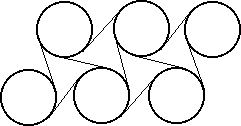Fig. 7 Chiral (noncentrosymmetric) hexagonal microstructure of rotatable nodes and bendable ligaments. Poisson's ratio is negative.

3. Role of generalized continuum mechanics

The non-affine, rotational and noncentrosymmetric degrees of freedom considered above in a structural sense can also be viewed in the context of continuum mechanics. Continuum theories for elastic materials are available with different amounts of freedom; the uniconstant and classical elasticity theories discussed above are the simplest examples. Although the continuum and structural views are distinct, we consider insight gained in one perspective to be transferable to the other.

Cosserat elasticity , also known as micropolar elasticity , allows the points in the solid rotational freedom as well as translational freedom. Correspondingly there is a couple per unit area or couple stress as well as the usual stress which is a force per unit area. An isotropic Cosserat solid is described by six elastic constants. Conceptually, this continuum representation corresponds to a microstructure containing rotatable elements which support bending or torsional loads  or are connected by ligaments which can support such loads. It had been suggested that Cosserat elasticity could be a mechanism for negative Poisson's ratios , however the range for Poisson's ratio in a Cosserat solid is the same as that for classical elasticity [32,34]. Cosserat solids differ from classical ones in that stress concentration factors differ from classical predictions, as does the rigidity of bars in bending and torsion. Such effects depend on the characteristic lengths which are additional elastic constants in Cosserat theory; if these lengths vanish classical elasticity is obtained as a special case. If the strains in the object vary over scales comparable to the characteristic lengths, then the stresses will differ significantly from classical predictions. Experiments have disclosed several materials to behave as Cosserat solids, but the Poisson's ratios are not unusual [see, e.g. ref. 35].

Chiral or noncentrosymmetric solids are those which are not invariant to an inversion of the coordinates. No mechanical effect of such asymmetry is predicted by classical elasticity. Cosserat elasticity allows the effects of chirality to be incorporated in a natural way; new effects are predicted such as the untwisting of a bar under tensile force, and size effects in Poisson's ratio .

Another generalized continuum theory is of elastic materials with microstructure, or micromorphic solids . This theory allows the points in the solid to translate, rotate, and deform; the theory is therefore more general than classical elasticity or Cosserat elasticity, and an isotropic solid is described by 18 elastic constants. The allowable range for Poisson's ratio is nevertheless identical to that for classical elastic solids . Since the local micro-deformation can differ from the macro-deformation, a microelastic solid of this type would experience non-affine deformation in the structural view.

Each structural mechanism considered here which gives rise to a negative Poisson's ratio is associated with a generalized continuum. Yet the generalized continuum theories allow the same range of Poisson's ratio as does classical elasticity. Not all solids which behave as generalized continua will have a negative Poisson's ratio, however all negative Poisson's ratio materials will be describable as generalized continua. The characteristic lengths may be sufficiently small that the resulting nonclassical effects are too small to observe. Nevertheless the Poisson's ratio is a macroscopic result of the microstructure; it is measurable no matter how small the structure is. For materials in which the structure size is sufficiently large, non-classical effects describable by generalized continuum mechanics can be substantial. It is important to consider which theory actually describes the material; for example Cosserat elasticity predicts a reduction of stress concentration factors for holes and cracks in comparison with classical values, while a different special case of microstructure elasticity predicts an increase [39,40].

4. Discussion

Several structural mechanisms have been found to give rise to a negative Poisson's ratio: non-affine deformation, non-central force interaction combined with prestrain, and chiral structure combined with either non-central force or non-affine deformation. The materials originally reported  by the author have freedom to undergo both non-affine deformation and bending of the ligaments (hence non-central force). Other materials which have been reported to have a negative Poisson's ratio are mostly anisotropic and exhibit the effect only in some directions; the mechanism of directional anisotropy has not been treated here. Rocks can be anisotropic, however it is possible that the effects reported in rocks with microcracks [8,9] are due to non-affine deformation in the opening of the microcracks. Theoretical analyses of the effect of microcracks  predict that when there are enough cracks to reduce the Poisson's ratio to zero, Young's modulus also becomes zero, so that negative Poisson's ratios are not predicted by these theories, and theory and experiment fail to agree in this case. Rocks can exhibit complex behavior: the Poisson's ratio of rock can depend very much on the stress history . Polymer gels represent another interesting class of materials: such gels can exhibit negative Poisson's ratios over a narrow temperature range during phase transitions . The mechanism for this has not been elucidated, however we surmise that prestrain is involved. It remains to be determined by experiment which structural mechanisms are most important in generating the effects in real materials such as the above.

Structural mechanisms are of interest in connection with the other material properties of materials with negative Poisson's ratios. For example, the non-affine mechanism is associated with an unfolding of the unit cells. Such unfolding results in a change in the relation between stress and strain under large deformation: the stress-strain curves for re-entrant foam tend to be more linear than those of conventional foams. As for dynamical aspects, the convoluted unit cells can vibrate and give rise to unusual acoustic behavior  not explicitly associated with the Poisson's ratio. Moreover, it is possible that non-affine deformation kinematics can be exploited to make new materials with unusual and useful acoustic behavior. As for density, the materials originally reported  were polymer foams of relatively low relative density (solid volume fraction) with cells of size 0.3 mm to 2.5 mm. The foam cells could in principle be arbitrarily small, down to molecular scale. The upper bound on the relative density of this type of material is not yet known, however we have no doubt there is such a bound and that it is significantly less than unity. As for stiffness and strength, low density in cellular solids is associated with low stiffness, and although metal foam is much stiffer than polymer foam [1,2], materials with negative Poisson's ratios with higher density would be of interest . The possibility of making high density 'solids' based on non-central forces with prestrain, or on chiral structures is an intriguing one. As for toughness and damage resistance, the Poisson's ratio itself has bearing upon the stress concentration factors in certain three-dimensional crack geometries  such that negative values of large magnitude are advantageous. The generalized continuum aspects of these materials also affect stress concentration factors. Consequently, the toughness and damage resistance of these materials is expected to depend on the deformation mechanisms described above and upon the cell size. Experiments are required to elucidate the connection.

Structural mechanisms are also of interest in connection with the fabrication of materials with negative Poisson's ratios. It is now known that materials can be made which undergo non-affine deformation and rib bending [1,2]. We have also made honeycombs based on the structures shown in Fig. 2 and 7. However many of the models which have been reported for materials with negative Poisson's ratios tend not to lend themselves to the making of materials. As for the present non-central force model with prestrain, it is possible that such prestrain could be introduced by selective crystallization and cooling. It remains to be seen whether materials with negative Poisson's ratios can be made in a controlled way based on the alternative models.

5. Conclusions

We conclude the following from this modelling study.

1. Negative Poisson's ratios can be obtained by a non-affine deformation geometry alone.

2. Negative Poisson's ratios can result from a combination of non-central forces and prestrain.

3. Negative Poisson's ratios can result from a chiral structure with rotational degrees of freedom combined with non-central force or non-affine deformation.

Top

References

1. R. S. Lakes, "Foam structures with a negative Poisson's ratio", Science , 235 (1987) 1038-1040

2. E.A. Friis, R.S. Lakes, and J.B. Park, "Negative Poisson's ratio polymeric and metallic materials", Journal of Materials Science, 23 (1988) 4406-4414

3. R.S. Lakes, "Foam materials with a negative Poisson's ratio," Developments in Mechanics, Vol. 14, p. 758-763, Proceedings, 20th Midwest Mechanics Conference, Purdue University, Aug. 31-Sept. 2, 1987

4 A. W. Lipsett and A. I. Beltzer, "Reexamination of dynamic problems of elasticity for negative Poisson's ratio", J. Acoust. Soc. Am 84, (1988) 2179-2186

5 A. Freedman, "The variation, with the Poisson's ratio, of Lamb modes in a free plate, I, general spectra", J. Sound and Vibration, 137, (1990) 209-230

6 C.P. Chen, and R.S. Lakes, "Dynamic wave dispersion and loss properties of conventional and negative Poisson's ratio polymeric cellular materials", Journal of Cellular Polymers, 8 (1989) 343-359

7 L. J. Gibson, M. F. Ashby, G. S. Schajer, and C. I. Robertson, "The mechanics of two dimensional cellular solids", Proc. Royal Society London, A382 (1982) 25-42

8 F. Homand-Etienne and R. Houpert, "Thermally induced microcracking in granites: characterization and analysis", Int. J. Rock Mech. Min. Sci & Geomech. Abstr. 26, 125-134, 1989

9 A. Nur and G. Simmons, "The effect of saturation on velocity in low porosity rocks", Earth and planetary science letters 7, (1969) 183-193

10 B.D. Caddock, and K.E. Evans, "Microporous materials with negative Poisson's ratio: I. Microstructure and mechanical properties, J. Phys. D., Appl. Phys. 22, (1989) 1877-1882

11 K.E. Evans, and B.D. Caddock, "Microporous materials with negative Poisson's ratio: II. Mechanisms and interpretation", J. Phys. D., Appl. Phys. 22, (1989) 1883-1887

12 C.T. Herakovich, "Composite laminates with negative through-the -thickness Poisson's ratio", J. Composite Materials 18, (1985) 447-455

13 Tsai, S.W. and Hahn, H.T., Introduction to composite materials, Technomic, Lancaster PA (1980)

14 M. Miki and Y. Morotsu, "The peculiar behavior of the Poisson's ratio of laminated fibrous composites", JSME International Journal, 32, (1989) 67-72

15 A. M. Garber, "Pyrolytic materials for thermal protection systems", Aerospace Engineering 22, (1963) 126-137

16 N. Bettenbouche, G. A. Saunders, E. F. Lambson, and W. Hönle, "The dependence of the elastic stiffness moduli and the Poisson ratio of natural iron pyrites FeS2 upon pressure and temperature", J. Phys. D., Appl. Phys. 22, (1989) 670-675

17 M. Ya. Popereka and V.G. Balagurov, "Ferromagnetic films having a negative Poisson's ratio", Soviet Physics Solid State 11, (1970) 2938-2943

18 R. F. Almgren, "An isotropic three dimensional structure with Poisson's ratio = -1", J. Elasticity, 15, (1985) 427-430

19 K.E. Evans, "Tensile network microstructures exhibiting negative Poisson's ratio", J. Phys. D., Appl. Phys. 22, (1989) 1870-1876

20 G. Simmons, and H. Wang, Single crystal elastic constants and calculated aggregate properties: a handbook, MIT Press, Cambridge, 2nd ed, 1971

21 J.H. Weiner, Statistical Mechanics of Elasticity, J. Wiley, NY, 1983

22 S. P. Timoshenko, History of Strength of Materials, Dover, NY, 1983

23 A.E.H. Love, A Treatise on the Mathematical Theory of Elasticity, 4th ed, Dover, NY, 1944

24 J. F. Nye, Physical Properties of Crystals, Oxford, 1976

25 L.J. Gibson and M. Ashby, Mechanics of cellular solids, Pergamon, 1988

26 A.G. Kolpakov, "On the determination of the averaged moduli of elastic gridworks", Prikl. Mat. Mekh 59, (1985) 969-977

27 K. Berglund, "Investigation of a two-dimensional model of a micropolar continuum", Archives of Mechanics 29, (1977) 383-392

28 R.J. Bathurst and L. Rothenburg, "Note on a random isotropic granular material with negative Poisson's ratio", Int. J. Engng. Sci, 26 (1988) 373-383

29 T.R. Tauchert, "A lattice theory for representation of thermoelastic composite materials", Recent Advances in Engineering Science 5, (1970) 325-345

30 K.W. Wojciechowski, "Two-dimensional isotropic system with a negative Poisson ratio", Physics Letters A, 137, (1989) 60-64

31 E. and F. Cosserat, Théorie des Corps Deformables, A. Hermann et Fils, 1909

32 A.C. Eringen, (1968) Theory of micropolar elasticity. In Fracture Vol. 1, 621-729

33 S. Burns, "Negative Poisson's ratio materials, letter", Science, 238, (1987) 551

34 R.S. Lakes, "Negative Poisson's ratio materials, reply", Science, 238, (1987) 551

35 Lakes, R.S., "Experimental microelasticity of two porous solids", International Journal of Solids and Structures, 22 (1986) 55-

36 R.S. Lakes and R. L. Benedict, "Noncentrosymmetry in micropolar elasticity", Int. J. Engng. Sci. 29, (1982) 1161-1167

37 R.D. Mindlin, "Microstructure in linear elasticity", Arch. Rational Mech. Anal. 16, (1964) 51-78

38 A.C. Eringen, "Mechanics of micromorphic continua", IUTAM symposium, Mechanics of Generalized Continua, E. Kröner, ed., Springer Verlag, 1968

39 J.L. Bleustein, "Effects of microstructure on the stress concentration at a spherical cavity", Int. J. Solids Structures 2, (1960) 83-104

40 S.C. Cowin, "The stresses around a hole in a linear elastic material with voids", Q. J. Mech. Appl. Math. 37 Pt. 3 (1984) 441-465

41 E.D. Case, "The effect of microcracking upon the Poisson's ratio for brittle materials", Journal of Materials Science 19, (1984) 3702-3712

42 O.G.Ingles, I.K. Lee, and R.C.Neil, "The influence of stress history on lateral strain", Rock Mechanics, 5, (1973) 203-213

43 S.Hirotsu, "Elastic anomaly near the critical point of volume phase transition in polymer gels", Macromolecules, 23, (1990) 903-905

44 J. Cherfas, "Stretching the point", Science 247, (1990) 630

Acknowledgment

Support of this research by the NSF, by the NASA/Boeing ATCAS program under contract #NAS1-18889, and by a University Faculty Scholar Award is gratefully acknowledged. Partial support by the ONR is also gratefully acknowledged. I thank Professor A. Ruina of Cornell University for his discussions on the subject of affine deformation and Professor J. B. Park of the University of Iowa for his critical review of the manuscript.

 Poisson main   Original article   Causal mechanisms   Chirality   Synthesis method   Names, auxetic materials, dilational materials, anti-rubber   Advances   Structural hierarchy   End effects   Fastener   Work by others
Top